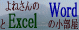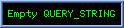よねさんのWordとExcelの小部屋Excel(エクセル)講座の総目次Excel(エクセル) VBA入門：目次｜条件分岐処理(If〜Then,Select Case)# Excel(エクセル) VBA入門：条件分岐処理(If〜Then,Select Case)

 スポンサードリンク

## 条件分岐

### If〜Then〜Else〜End Iftopへ

• 条件によって異なったステートメントを実行します。
1. セル範囲B1:B5で値が
1の時は赤色、2の時は黄色、3の時は水色、それ以外の時は緑色
でセルを塗りつぶします。
•  Sub rei_01() 　Dim myRng As Range 　Dim c As Range 　Dim myColor As Integer 　Set myRng = Range("B1:B5") 　　For Each c In myRng 　　　　If c.Value = 1 Then 　　　　　　myColor = 3 '赤 　　　　ElseIf c.Value = 2 Then 　　　　　　myColor = 6 '黄 　　　　ElseIf c.Value = 3 Then 　　　　　　myColor = 8 '水色 　　　　Else 　　　　　　myColor = 10 '緑 　　　　End If 　　　　　　c.Interior.ColorIndex = myColor 　　Next c End Sub
• 実行結果### Select Case〜End Selecttopへ

• 条件によって異なったステートメントを実行します。
1. セル範囲B1:B5で値が
1の時は赤色、2の時は黄色、3の時は水色、それ以外の時は緑色
でセルを塗りつぶします。
•  Sub rei_02() 　Dim myRng As Range 　Dim c As Range 　Dim myColor　As　Integer 　Set myRng = Range("B1:B5") 　　For Each c In myRng 　　　　Select Case c.Value 　　　　　　Case 1 　　　　　　　　myColor = 3 '赤 　　　　　　Case 2 　　　　　　　　myColor = 6 '黄 　　　　　　Case 3 　　　　　　　　myColor = 8 '水色 　　　　　　Case Else 　　　　　　　　myColor = 10 '緑 　　　　End Select 　　　　c.Interior.ColorIndex = myColor 　　Next c End Sub
• 実行結果2. 条件範囲の書き方1　[条件がある値またはある値といったOR条件の場合]
• セル範囲B1:B6で値が
1と3の時は赤色、2と4の時は黄色、5の時は水色、それ以外の時は緑色に
(1または3の時は赤色、2または4の時は黄色、5の時は水色、それ以外の時は緑色に　と書いた方が分かりやすいかもしれません)
セルを塗りつぶします。
•  Sub rei_03() 　Dim myRng As Range 　Dim c As Range 　Dim myColor　As　Integer 　Set myRng = Range("B1:B6") 　　For Each c In myRng 　　　　Select Case c.Value 　　　　　　Case 1, 3 　　　　　　　　myColor = 3 '赤 　　　　　　Case 2, 4 　　　　　　　　myColor = 6 '黄 　　　　　　Case 5 　　　　　　　　myColor = 8 '水色 　　　　　　Case Else 　　　　　　　　myColor = 10 '緑 　　　　End Select 　　　　c.Interior.ColorIndex = myColor 　　Next c End Sub
• 実行結果3. 条件範囲の書き方2　[条件がある範囲で、比較演算子を使用する場合]
• セル範囲B1:B5で値が
10未満の時は赤色、20未満の時は黄色、30未満の時は水色、それ以外の時は緑色
でセルを塗りつぶします。
•  Sub rei_04() 　Dim myRng As Range 　Dim c As Range 　Dim myColor　As　Integer 　Set myRng = Range("B1:B5") 　　For Each c In myRng 　　　　Select Case c.Value 　　　　　　Case Is < 10 　　　　　　　　myColor = 3 '赤 　　　　　　Case Is < 20 　　　　　　　　myColor = 6 '黄 　　　　　　Case Is < 30 　　　　　　　　myColor = 8 '水色 　　　　　　Case Else 　　　　　　　　myColor = 10 '緑 　　　　End Select 　　　　c.Interior.ColorIndex = myColor 　　Next c End Sub
• 実行結果4. 条件範囲の書き方3　[条件がある範囲である場合]
• セル範囲B1:B5で値が
1以上9以下の時は赤色、10以上19以下の時は黄色、20以上29以下の時は水色、それ以外の時は緑色
でセルを塗りつぶします。
• この例では　c.Valueは整数値であることを想定していますので、9.5は範囲外とされ緑で塗りつぶされます。
•  Sub rei_05() 　Dim myRng As Range 　Dim c As Range 　Dim myColor As Integer 　Set myRng = Range("B1:B5") 　　For Each c In myRng 　　　　Select Case c.Value 　　　　　　Case 1 To 9 　　　　　　　　myColor = 3 '赤 　　　　　　Case 10 To 19 　　　　　　　　myColor = 6 '黄 　　　　　　Case 20 To 29 　　　　　　　　myColor = 8 '水色 　　　　　　Case Else 　　　　　　　　myColor = 10 '緑 　　　　End Select 　　　　c.Interior.ColorIndex = myColor 　　Next c End Sub
• 実行結果スポンサードリンク

よねさんのWordとExcelの小部屋Excel(エクセル)講座の総目次Excel(エクセル) VBA入門：目次｜条件分岐処理(If〜Then,Select Case)

PageViewCounterSince2006/2/27